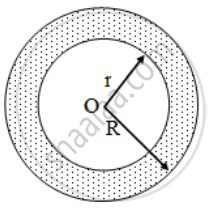# A race track is in the form of a ring whose inner circumference is 352 m, and the outer circumference is 396 m. Find the width of the track. - Mathematics

Sum

A race track is in the form of a ring whose inner circumference is 352 m, and the outer circumference is 396 m. Find the width of the track.

#### Solution

Let the outer and inner radii of the ring be R metres and r metres respectively. Then,2πR = 396 and 2πr = 352

⇒ 2 × \frac { 22 }{ 7 } × R = 396 and 2 × \frac { 22 }{ 7 } × r = 352

⇒ R = 396 × \frac { 7 }{ 22 } × \frac { 1 }{ 2 } and r = 352 × \frac { 7}{ 22 } × \frac { 1 }{ 2 }

⇒ R = 63 m and r = 56 m

Hence, width of the track = (R – r) metres

= (63 – 56) metres = 7 metres

Concept: Circumference of a Circle
Is there an error in this question or solution?

Share